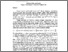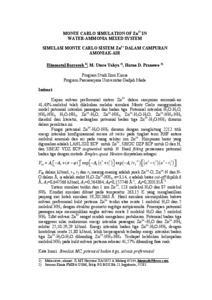# Simulasi monte carlo sistem Zn2+ dalam campuran amoniak air Monte carlo simulation of Zn2+ in water ammonia mix system

Barroroh, Himmatul and Yahya, M. Utoro and Pranowo, Harno D. (2004) Simulasi monte carlo sistem Zn2+ dalam campuran amoniak air Monte carlo simulation of Zn2+ in water ammonia mix system. Sains dan Sibernatika, XVII (1).Preview
Text
simulasi.pdf

Full text available at: http://i-lib.ugm.ac.id/jurnal/detail.php?dataId=44...

## Abstract

Monte Carlo Simulation of Zn2+ in 41.43 mol% water-ammonia mixed system was carried out using pair potential including three-body potential in order to study preferential solvation system. Interaction potential of H20-H20, NH3-NH3, H2O-NH3, Zn2+-H20, Zn2+-NH3, Zn2+-H2O-H20, Zn2+-NH3-NH3 were adopted from literature while three-body potential of Zn2+- H2O-NH3 was constructed in this study.
Potential function of Zn2+-H2O-NH3 was constructed by collecting 2212 interaction energy points between ammonia and water molecules around Zn2+ ion. The energy of the system was calculated by ab initio method at RHF level of theory using LANL2DZ ECP basis set for Zn2+, SBKJC DZP ECP for 0 and H atoms, and SBKJC VDZ ECP augmented for N atom. Fitting function result of three-body potential by Simplex-quasi Newton method was written as:
V3b = A 1[â€“ + â€“ cp exp[â€“A3 (r12 + r22)]
exp (â€“A4 / r3-2 )I[(a2 â€“r12)(a2â€“r22)]
where V3b in kJ/ mol, rl, r2 and r3 were distance (A) of Zn2+-0, Zn2+-N, and N-0 respectively, cp is H20-Zn2+-NH3 angle in radian, n=3.14, a is cut-off limit set as 6 A, A1=0.647566 kJ/mol, A2=0.564364, A3=0.155746 A-2, A4=0.203131A-2.
Simulation system consisted of 1 ion Zn2+, 123 H2O molecules, and 87 NH3 molecules. Temperature of the system was set at 263.15 K resulted simulation box lenght of 19.2012663 A. The result showed that first solvation shell of Zn2+ consist of average 1 H2O molecule and 5 ammonia molecules withp first potential only eometr led to
of trigonal antipyramida. Appl g y structure
ying pair
solvation shell of average 3 H20 molecules and 5 NH 3
Ligand exchange reaction was particularly easy to occur molecul between
es.
first and second solvation sphere of Zn2+. i are
energy enerand
Thmum of Zn2+-H2O potential otential
affects to shift pair interaction energy max
Zn2+-NH3 about 25.10-29.29 kr/mol. Three-body ptential of Zn2+-H2O-NH3 with eve age contribution of o 21.80 favourably affects three-body potential of Zn2+- kJ/mol
kli
H2O-H20 comp
with Zn2+-NH3-NH3. There was an excess amount of NI-I
first solvation shell about 41.57% compare with the bulk. 3 in the

Item Type: Journal Article MC simulation, three-body potential, preferential solvation 03 CHEMICAL SCIENCES > 0302 Inorganic Chemistry > 030207 Transition Metal Chemistry03 CHEMICAL SCIENCES > 0306 Physical Chemistry (incl. Structural) > 030606 Structural Chemistry and Spectroscopy03 CHEMICAL SCIENCES > 0307 Theoretical and Computational Chemistry > 030799 Theoretical and Computational Chemistry not elsewhere classified Faculty of Mathematics and Sciences > Department of Chemistry Himmatul Barroroh 26 Jan 2017 19:20View Item